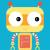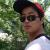## 在Java中使用Math.round语句

Javenty 发布于 2015/05/12 00:00

Math.round(10*(Math.sqrt(20)+Math.sqrt(10))/(Math.sqrt(20)-Math.sqrt(10)))/10.0与

Math.round(10*(Math.sqrt(20)+Math.sqrt(10))/(Math.sqrt(20)-Math.sqrt(10)))/10的结果为什么一个是5.8一个是5

0Jacenty，我想你应该明白Java中的自动转型，对不？ 那我们今天就拿你的问题来分析下吧

知识点： 数据类型精度比较   int(整型) < double(双精度) 或者 float(浮点型)

首先 你知道 Math.round(10*(Math.sqrt(20)+Math.sqrt(10))/(Math.sqrt(20)-Math.sqrt(10))) 返回的是一个Int类型的，我们常常称为整数型，而 10.0 我们暂时认为 double 吧，所以在Java 的自动转型中有一套规则 就是按最大精度返回结果，所以返回的是 double 类型，而当除以10的时候，因为都是整数，所以失去了小数部分的精度。

希望对你有帮助，望采纳

0float 与 int的区别